# Local limit theorems

(diff) ← Older revision | Latest revision (diff) | Newer revision → (diff)

in probability theory

Limit theorems for densities, that is, theorems that establish the convergence of the densities of a sequence of distributions to the density of the limit distribution (if the given densities exist), or a classical version of local limit theorems, namely local theorems for lattice distributions, the simplest of which is the local Laplace theorem.

Letbe a sequence of independent random variables that have a common distribution functionwith meanand finite positive variance. Let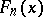be the distribution function of the normalized sum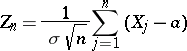and let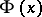be the normal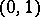-distribution function. The assumptions ensure that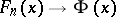asfor any. It can be shown that this relation does not imply the convergence of the density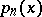of the distribution of the random variable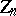to the normal density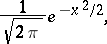even if the distributionhas a density. If, for some, has a bounded density, then(*)

uniformly with respect to. The condition that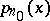is bounded for someis necessary for (*) to hold uniformly with respect to.

Let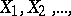be a sequence of independent random variables that have the same non-degenerate distribution, and suppose that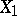takes values of the form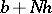,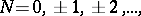with probability 1, whereandare constants (that is,has a lattice distribution with step).

Suppose thathas finite variance, let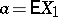and let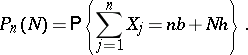In order that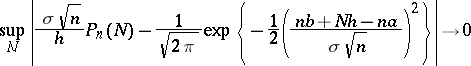asit is necessary and sufficient that the stepshould be maximal. This theorem of B.V. Gnedenko is a generalization of the local Laplace theorem.

Local limit theorems for sums of independent non-identically distributed random variables serve as a basic mathematical tool in classical statistical mechanics and quantum statistics (see , ).

Local limit theorems have been intensively studied for sums of independent random variables and vectors, together with estimates of the rate of convergence in these theorems. The case of a limiting normal distribution has been most fully investigated (see , Chapt. 7); a number of papers have been devoted to local limit theorems for the case of an arbitrary stable distribution (see ). Similar investigations have been carried out for sums of dependent random variables, in particular for sums of random variables that form a Markov chain (see , ).

How to Cite This Entry:
Local limit theorems. Encyclopedia of Mathematics. URL: http://encyclopediaofmath.org/index.php?title=Local_limit_theorems&oldid=11793
This article was adapted from an original article by V.V. Petrov (originator), which appeared in Encyclopedia of Mathematics - ISBN 1402006098. See original article